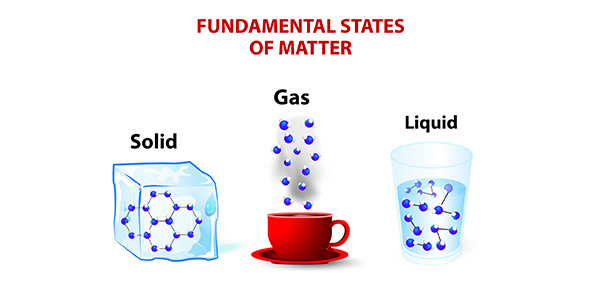# Chemistry Chapters 3 & 4: Matter

22 QuestionsSettingsRelated Topics
• 1.
What did Dalton's atomic theory of matter state?
• 2.
Is neither created nor destroyed, only changed. What is it and what states this?
• A.

Matter; Law of Matter

• B.

Mass; Law of Conservation of Mass

• C.

Matter; Law of Conservation of Matter

• D.

Mass; Law of Constitution of Mass

• 3.
We measure elements and compounds by their...
• A.

Ratios of mass

• B.

Ratios of matter

• C.

Average Atomic Mass

• D.

EM Waves

• 4.
What states: The elements that compose the compounds are always in a certain proportion by mass.
• A.

Law of conservation of elements

• B.

Law of conservation of compounds

• C.

Law of definite proportions

• D.

Law of indefinite proportions

• 5.
Who proposed his atomic theory of matter in 1803?
• 6.
Atoms of the element that are chemically alike but differ in mass. (Same element, different number of neutrons.)
• 7.
The sum of the protons and the neutrons=
• 8.
EM Waves include:
• A.

Energy

• B.

Sound

• C.

Light

• D.

TV

• E.

Microwave

• F.

Speed

• 9.
Order of electrons in the main four energy levels:
• A.

1st=2; 2nd=4; 3rd=8; 4th=10

• B.

1st-1; 2nd-5; 3rd-15; 4th-31

• C.

1st-2; 2nd-8; 3rd-16; 4th-30

• D.

1st-2; 2nd-8; 3rd-18; 4th-32

• 10.
Electrons in the outermost energy level.
• 11.
The magic number in chemistry is....
• 12.
Uses dots to represent valence electrons: A ______ ____ Diagram
• 13.
Choose the correct way to calculate atomic mass:
• A.

Mass contribution*mass of isotope=abundance

• B.

Mass contribution=mass of isotope*abundance

• C.

Mass contribution*abundance=mass of isotope

• 14.
The average atomic mass is the sum of the...
• A.

Mass contributions of each isotope.

• B.

Mass of each isotope.

• C.

Abundance of each isotope.

• D.

Mass contributions and abundance of each isotope.

• 15.
Chemical or physical: You CANNOT reverse it.
• 16.
The Law of Definite proportions is measured by:
• A.

Ratio of Mass

• B.

Proportions of Mass

• C.

Percent by Mass

• D.

Proportions of Matter

• 17.
mass of element ______________                  x            100    =      ? mass of compound
• 18.
States the different masses of one element combine with the same relative masses of another element to form a different compound.